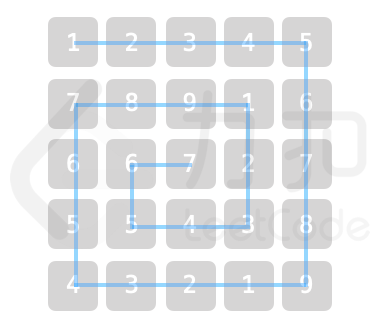# leetcode 2021 春季编程大赛

## 零、背景

2021年04月05日，参加了 lleetcode 2021 春季编程大赛个人赛。

https://leetcode-cn.com/contest/season/2021-spring/problems/4xy4Wx/

https://leetcode-cn.com/contest/season/2021-spring/ranking/solo/

https://github.com/tiankonguse/leetcode-solutions/tree/master/season/2021-spring/person

## 一、采购方案

``````int purchasePlans(vector<int>& nums, int target) {
ll ans = 0;
sort(nums.begin(), nums.end());

int n = nums.size();
for(int i=0;i<n;i++){
if(nums[i] >= target) break;

int dis = target - nums[i];

auto it = std::upper_bound (nums.begin(), nums.end(), dis);
int num = std::distance(nums.begin(), it);
if(i < num){ // 不能选自己，减去一个
ans += num - 1;
}else{
ans += num;
}
}

return ans/2 % 1000000007;
}
``````

``````for (int j=n-1,i=0;i<n;i++) {
for (;j>i && a[i]+a[j]>target;--j);
if (j<=i) break;
ret=(ret+j-i)%MOD;
}
``````

## 二、乐团站位PS：这道题由于是由原先模拟一圈修改成模拟去掉 k 圈，很多地方没修改过来，WA 了无数次。

``````class Solution {
ll mymin(ll a, ll b) { return a < b ? a : b; }
int solver(ll n, ll x, ll y, ll start) {
ll lev = n; // 边界，可以去掉几圈
lev = mymin(lev, x - 0 + 1);
lev = mymin(lev, n - x);
lev = mymin(lev, y - 0 + 1);
lev = mymin(lev, n - y);
lev = lev - 1;

// 等差数列，计算出偏移量
// 4 * (n-1) + 4 * (n-2) + .. + 4 * (n - lev)
ll pos = 4 * ((n - 1) + (n - 1 - (lev - 1) * 2)) * lev / 2;
start = (start + pos) % 9;
x -= lev; //坐标修正
y -= lev;
n -= lev * 2; //边长修正

if (x == 0 || y + 1 == n) {  // 上
return (start + x + y) % 9;
}
if (x + 1 == n || y == 0) {  // 下
return (start + 4 * (n - 1) - y - x) % 9;
}
return 0;
}

public:
int orchestraLayout(int num, int x, int y) {
return solver(num, x, y, 0) + 1;
}
};
``````

## 三、魔塔游戏

``````int magicTower(vector<int>& nums) {
min_queue<ll> que; //维护一个最小堆
ll sum = 1;
int ans = 0;
ll left = 0;
for(auto v: nums){
if(v == 0) continue;
if(v < 0){
que.push(v);
}
sum += v;

if(sum >= 0) continue;

sum -= que.top();
left += que.top();
que.pop();
ans++;
}
return sum + left > 0 ? ans : -1;
}
``````

## 五、批量处理任务## 六、最后

《完》

-EOF-

QQ算法群：165531769（不止算法）

## 关注公众号,接收最新消息

1.## 关注小密圈,学习各种算法

1.